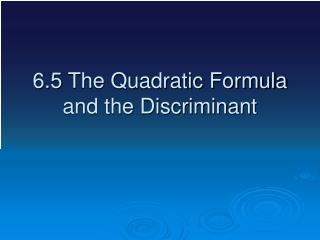DownloadDownload Presentation6.5 The Quadratic Formula and the Discriminant

# 6.5 The Quadratic Formula and the Discriminant

Download Presentation## 6.5 The Quadratic Formula and the Discriminant

- - - - - - - - - - - - - - - - - - - - - - - - - - - E N D - - - - - - - - - - - - - - - - - - - - - - - - - - -
##### Presentation Transcript

1. 6.5 The Quadratic Formula and the Discriminant

2. We have a number of different way of finding the roots if a quadratic equations #1. Making a table #2. Factoring #3. Completing the Square Now a new way that comes from completing the square. The Quadratic Formula

3. The Quadratic Formula Solve for x by completing the square.

4. The Quadratic Formula Solve for x by completing the square.

5. The Quadratic Formula Solve for x by completing the square.

6. The Quadratic Formula Solve for x by completing the square.

7. Yes, you can remember this formula Pop goes the Weasel http://www.youtube.com/watch?v=2lbABbfU6Zc&feature=related Gilligan’s Island http://www.youtube.com/watch?v=3CWTt9QFioY&feature=related This one I can’t explain http://www.youtube.com/watch?v=haq6kpWdEMs&feature=related

8. How does it work Equation:

9. How does it work Equation:

10. The Discriminant The number in the square root of the quadratic formula.

11. The Discriminant The Discriminant can be negative, positive or zero If the Discriminant is positive, there are 2 real answers. If the square root is not a prefect square ( for example ), then there will be 2 irrational roots ( for example ).

12. The Discriminant The Discriminant can be negative, positive or zero If the Discriminant is positive, there are 2 real answers. If the Discriminant is zero, there is 1 real answer. If the Discriminant is negative, there are 2 complex answers. complex answer have i.

13. Solve using the Quadratic formula

14. Solve using the Quadratic formula

15. Solve using the Quadratic formula

16. Solve using the Quadratic formula

17. Solve using the Quadratic formula

18. Solve using the Quadratic formula

19. Solve using the Quadratic formula

20. Solve using the Quadratic formula

21. Solve using the Quadratic formula

22. Solve using the Quadratic formula

23. Solve using the Quadratic formula

24. Describe the roots Tell me the Discriminant and the type of roots

25. Describe the roots Tell me the Discriminant and the type of roots 0, One rational root

26. Describe the roots Tell me the Discriminant and the type of roots 0, One rational root

27. Describe the roots Tell me the Discriminant and the type of roots 0, One rational root -11, Two complex roots

28. Describe the roots Tell me the Discriminant and the type of roots 0, One rational root -11, Two complex roots

29. Describe the roots Tell me the Discriminant and the type of roots 0, One rational root -11, Two complex roots 80, Two irrational roots

30. Homework Page 318 # 15 – 39 odd

31. Homework Page 318 # 14 – 38 even # 61 - 66